# Scale of the map

Determine the scale of the map if the actual distance between A and B is 720 km and the distance on the map is 20 cm.

M =  3600000

### Step-by-step explanation: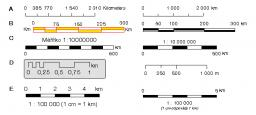Did you find an error or inaccuracy? Feel free to write us. Thank you!Tips to related online calculators
Do you want to convert length units?

## Related math problems and questions:

• Scale of the mapDetermine the map's scale, which is the actual distance of 120 km l represented by a segment long 6 cm.
• The scaleThe distance of 15 cm on the map corresponds to a distance of 27 km in reality. What is the scale of the map?
• Map scaleGarden has on plan on a scale of 1: 150 width 22 cm and length 35 cm. What is the real area of the garden?
• Scale of the map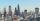The distance between two cities is actually 30 km and the map is 6 cm. What is the scale of the map?
• A map 3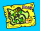A map is drawn to a scale of 1 : 25 000. This scale can be expressed as 1 cm represents n km. Find n.
• Map 2At what scale is made map if the distance 8.2 km corresponds on the map segment 5 cm long?
• Central Park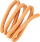Central Park is a rectangular park in New York City and the map scale is 1 cm: 320 m (It means 1 cm on the map represents 320 m in the real world. ). Find the north-south distance if the length on the map is 2.5 cm.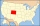The state Colorado has the shape of a rectangle. The map is sold on 2 posters. The first has dimensions of 70 cm and 50 cm in a scale of 1: 1,000,000. The second poster has dimensions of 175 cm and 125 cm. What is the scale of the second poster?
• On the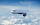On the map of Europe made at 1: 4000000, Bratislava and Paris' distance is 28 cm. At what time an airplane flying 800 km/h will fly this journey?
• Two villages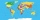Two villages are 11 km and 500 m away. On the map, their distance is determined by a 5 cm long line. Find the scale of the map.
• Map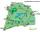On the tourist map at a scale of 1 : 50000 is distance between two points along a straight road 3.7 cm. How long travels this distance on a bike at 30 km/h? Time express in minutes.
• Two monuments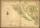The distance between two historical monuments on a map with a scale of 1: 500000 is 48 mm. Find the actual distance between these monuments in km.
• Image scale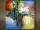The actual image dimensions are 60 cm x 80cm and has a reduced size 3 cm x 4 cm. At what scale the image was reduced?
• Map scale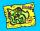What is the scale of the map, if 5.4 km is 72 mm on the map?
• Two citiesOn a map, 1.5 cm represents 50 miles. If the distance between the two cities on the map is 6 cm, how many miles separate the cities?
• Plan of the villagePlan of the municipality in 1:1000 scale has plotted garden with dimensions 25 mm and 28 mm. Determine the area of gardens in ares in reality.
• On the mapA line 1.5 cm long corresponds to a line 3 cm long on the map. What is the scale of the map?